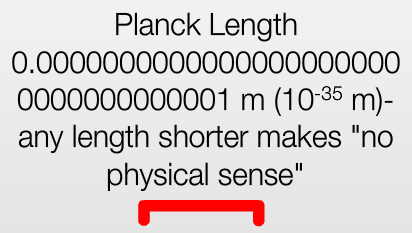A physics meme is circulating on the Interwebs, suggesting that any length shorter than the so-called Planck length makes “no physical sense”.

Which, of course, is pure nonsense.

The Planck length is formed using the three most fundamental constants in physics: the speed of light, $$c = 3\times 10^8~{\rm m}/{\rm s}$$; the gravitational constant, $$G = 6.67\times 10^{-11}~{\rm m}^3/{\rm kg}\cdot{\rm s}^2$$; and the reduced Planck constant, $$\hbar = h/2\pi = 1.05\times 10^{-34}~{\rm m}^2{\rm kg}/{\rm s}$$.

Of these, the speed of light just relates two human-defined units: the unit of length and the unit of time. Nothing prevents us from using units in which $$c = 1$$; for instance, we could use the second as our unit of time, and the light-second ($$= 300,000~{\rm km}$$) as our unit of length. In other words, the expression $$c = 300,000,000~{\rm m}/{\rm s}$$ is just an instruction to replace every occurrence of the symbol $${\rm s}$$ with the quantity $$300,000,000~{\rm m}$$.

If we did this in the definition of $$G$$, we get a new value: $$G’ = G/c^2 = 7.41\times 10^{-28}~{\rm m}/{\rm kg}$$.

Splendid, because this reveals that the gravitational constant is also just a relationship between human-defined units: the unit of length vs. the unit of mass. It allows us to replace every occurrence of the symbol $${\rm kg}$$ with the quantity $$7.41\times 10^{-28}~{\rm m}$$.

So let’s do this to the reduced Planck constant: $$\hbar’ = \hbar G/c^3 = 2.61\times 10^{-70}~{\rm m}^2$$. This is not a relationship between two human-defined units. This is a unit of area. Arguably, a natural unit of area. Taking its square root, we get what is called the Planck length: $$l_P = 1.61\times 10^{-35}~{\rm m}$$.

The meme suggests that a distance less than $$l_P$$ has no physical meaning.

But then, take two gamma rays, with almost identical energies, differing in wavelength by one Planck length, or about $$10^{-35}~{\rm m}$$.

Suppose these gamma rays originate from a spacecraft one astronomical unit (AU), or about $$1.5\times 10^{11}~{\rm m}$$ from the Earth.

The wavelength of a modest, $$1~{\rm MeV}$$ gamma ray is about $$1.2\times 10^{-12}~{\rm m}$$.

The number of full waves that fit in a distance of $$1.5\times 10^{11}~{\rm m}$$ is, therefore, is about $$1.25\times 10^{23}$$ waves.

A difference of $$10^{-35}~{\rm m}$$, or one Planck length, in wavelength adds up to a difference of $$1.25\times 10^{-12}~{\rm m}$$ over the $$1~{\rm AU}$$ distance, or more than one full wavelength of our gamma ray.

In other words, a difference of less than one Planck length in wavelength between two gamma rays is quite easily measurable in principle.

In practice, of course we’d need stable gamma ray lasers placed on interplanetary spacecraft and a sufficiently sensitive gamma ray interferometer, but nothing in principle prevents us from carrying out such a measurement, and all the energy, distance, and time scales involved are well within accessible limits at present day technology.

And if we used much stronger gamma rays, say at the energy level of the LHC (which is several million times more powerful), a distance of only a few thousand kilometers would be sufficient to detect the interference.

So please don’t tell me that a distance less than one Planck length has no physical meaning.